Home > A2C > Chapter 8 > Lesson 8.1.2 > Problem8-20

8-20.
1. Complete the table of values for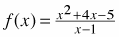. 8-20 HW eTool (Desmos). Homework Help ✎

 x −2 −1 0 1 2 3 y
1. Graph the points in the table. What kind of function does it appear to be? Why is it not correct to connect all of the dots?

2. Look for a simple pattern for the values in the table. What appears to be the relationship between x and y? Calculate f (0.9) and f (1.1) and add the points to your graph. Is there an asymptote at x = 1? If you are unsure, calculate f (0.99) and f (1.01) as well.

3. Simplify the formula for f(x). What do you think the complete graph looks like?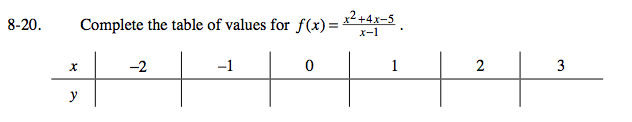Doing the substitutions yourself will help you understand what is happening in this problem.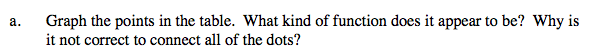Notice that there is no point at x = 1.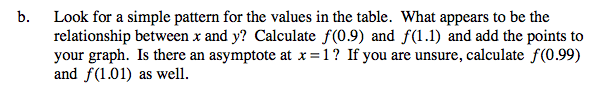The relationship between x and y appears to be linear. f(0.9) = 5.9, f(1.1) = 6.1.
Although the line is approaching the point (1, 6) from both sides, a point is not an asymptote.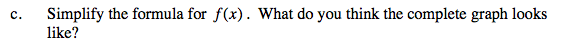$f(x) = \frac{(x + 5)(x - 1)}{x - 1} = x + 5$

Use the eTool below to complete the table.
Click the link at right for the full version of the eTool: A2C 8-20 HW eTool